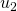# Introduction to Arithmetic Sequences

Definition: An arithmetic sequence is an ordered list – usually of numbers – where there is a common difference between terms.

Here is an example:Here is a second example:Notice that in these two examples, the common difference between terms is 3.

If you continue these sequences indefinitely, will there ever be a common term?

Create two arithmetic sequences such that there are common terms. Write out several terms of each sequence. Circle the terms that appear in both sequences. How far apart are they in each sequence? Why?

On the next applet, 3 of the first 7 terms of an arithmetic sequence are presented. Figure out the missing terms.

What method did you use to calculate the missing terms?

On these problems, two of the first seven terms of an arithmetic sequence are given. Figure out the first term.

What method did you use to find the first term?

Suppose we label the first term, the second term, and the seventh term. Figure out an expression forusingand. That is, figure out a step by step process that works every time you hit ‘new problem’ on this applet.